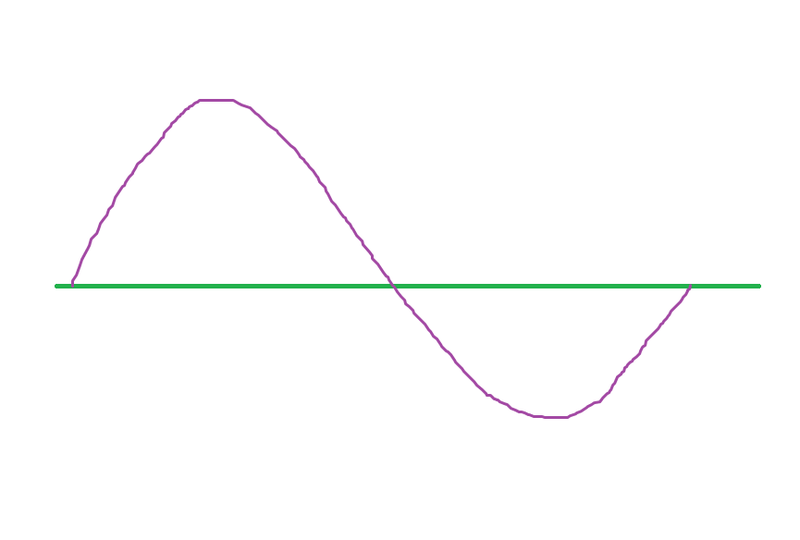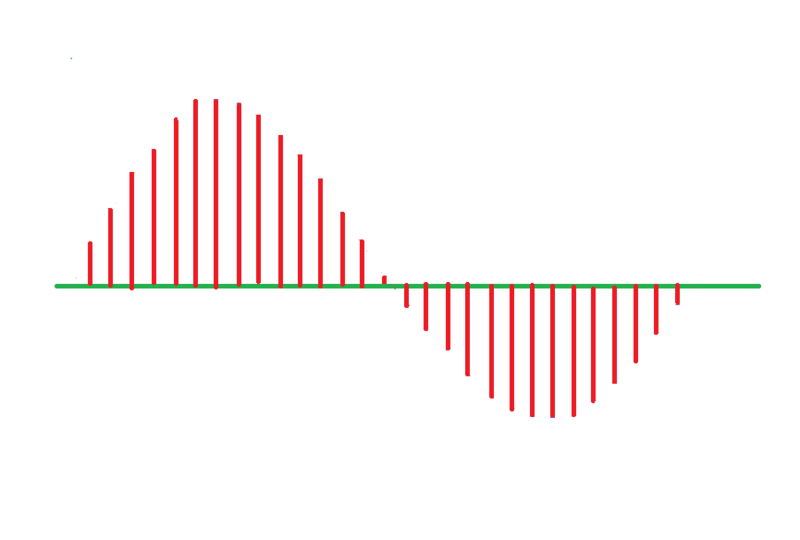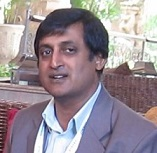Date: 04/04/2022

# Speech/voice recognition: Digitizing voice/audio signal

In the previous "Audio Signal Properties" we have studied the electrical properties of audio signal. In the world of digital electronics, the semiconductor logic and processing devices does not compute without digitizing the analog signal. A semiconductor device called analog to digital converter is used to convert linearly varying analog-flow into multi-bit digital binary numbers of where constant output voltage of 5V/3.3V is binary number 1 and zero voltage is binary number 0, representing amplitude quantity of analog signal. To give an example, a 6 volt measuring analog signal can have 8-bit binary output of 00000110. I will not discuss here how ADC circuit of semiconductor transistor/MOSFETs convert analog signal into digital. Instead we focus on aspects of quantizing, sampling, and digital representation of analog data.

Lets take a sample single cycle of an audio signal which looks like as shown in the Pic number-1 below. Due to limitations of present analog to digital converters, you can not convert every instant of analog signal into digital signal. Some instance or sample of analog signal is taken in such a periodic way that the space between samples is decided in such a way that the difference in amplitude of adjacent samples is very minimal. That rate of sampling is decided by a law called Nyquist Law. Nyquist Law is very simple its states that the frequency of sampling should be two times the maximum frequency of analog signal. Since maximum frequency of audio signal is 20 Kilo Hertz, ideally the sampling frequency should be 40 kilo Hertz.Pic number-1
The Pic number 2 below tells how a sampled signal looks.Pic number-2

If we construct back the original signal from the sampled pulse using digital to analog converter and filter, the constructed signal is as good as original signal except for the steps of sudden and very small incremental voltage rise and fall, which introduce a noise called quantization noise. Pic number-3 shows reconstructed audio signals.Pic number-3

So once the analog signal is sampled and quantized. The digitalization now involved lot of software to digitize, compress and secure the data. We will talk about that in our next module.

Read the 1st module: Speech/voice recognition: Electrical properties of audio signal

Author: Srinivasa Reddy N